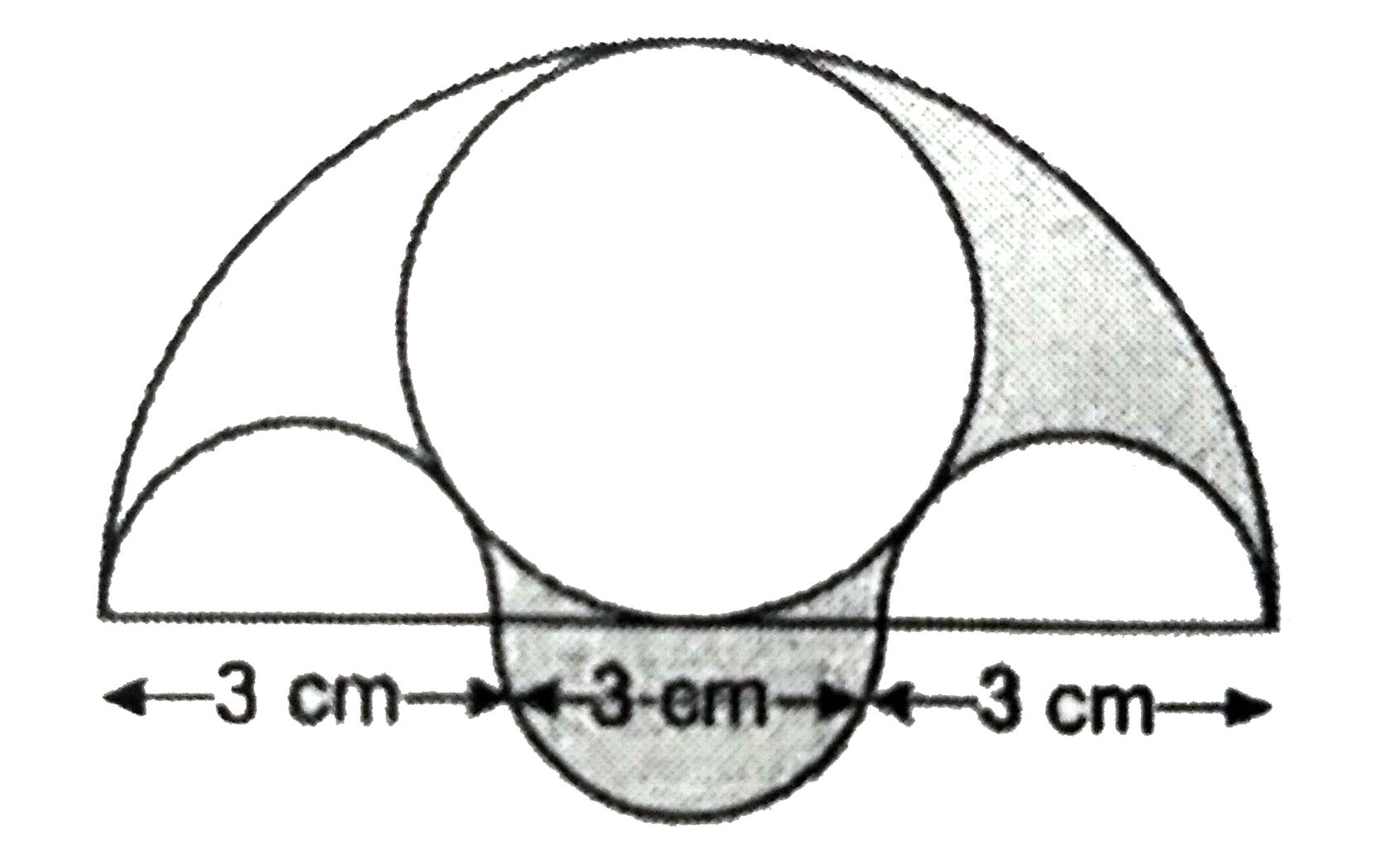# Three semicircles each of diameter 3 cm, a circle of diameter 4.5 cm and a semicircle of radius 4.5 cm are drawn in the adjoining figure. Find the area of the shaded region.##### Updated On: 27-06-2022

Text Solution

Solution
Required area = (area of large semicircle with radius 4.5 cm)
(area of two smaller semicircle with radius of each 32cm and a circle with radius 4.52cm) + (area of smaller semicircle with radius 32cm)
=12π(4.5)2[2×12π(32)2+π(4.52)2]+[12π(32)2]
=12π(4.5)2π(32)2π(4.52)2+12π(32)2
=14π[2×(4.5)29(4.5)2+92]=14π×4.5[2×4.524.5+1]
=14π×4.5(3.5)=14×227×4.5×3.5=12.375cm2